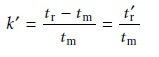Home | | Modern Analytical Chemistry | Capacity Factor - Theory of Column Chromatography

# Capacity Factor - Theory of Column Chromatography

The distribution of a solute, S, between the mobile phase and stationary phase can be represented by an equilibrium reaction

Capacity Factor

The distribution of a solute, S, between the mobile phase and stationary phase can be represented by an equilibrium reaction

Sm < = = = = > Ss

and its associated partition coefficient, KD, and distribution ratio, D,12.2

where the subscripts m and s refer to the mobile phase and stationary phase, respec- tively. As long as the solute is not involved in any additional equilibria in either the mobile phase or stationary phase, the equilibrium partition coefficient and the dis- tribution ratio will be the same.

Conservation of mass requires that the total moles of solute remain constant throughout the separation, thus

(moles S)tot = (moles S)m + (moles S)s    â€¦â€¦â€¦â€¦..12.3

Solving equation 12.3 for the moles of solute in the stationary phase and substitut- ing into equation 12.2 giveswhere Vm and Vs are the volumes of the mobile and stationary phases. Rearranging and solving for the fraction of solute in the mobile phase, fm, givesNote that this equation is identical to that describing the extraction of a solute in a liquidâ€“liquid extraction. Since the volumes of the sta- tionary and mobile phase may not be known, equation 12.4 is simplified by dividing both the numerator and denominator by Vm; thusis the soluteâ€™s capacity factor.

A soluteâ€™s capacity factor can be determined from a chromatogram by measur- ing the columnâ€™s void time, tm, and the soluteâ€™s retention time, tr (see Figure 12.7). The mobile phaseâ€™s average linear velocity, u, is equal to the length of the column, L, divided by the time required to elute a nonretained solute.12.7By the same reasoning, the soluteâ€™s average linear velocity, v, is12.8

The solute can only move through the column when it is in the mobile phase. Its average linear velocity, therefore, is simply the product of the mobile phaseâ€™s aver- age linear velocity and the fraction of solute present in the mobile phase.

v = ufm â€¦â€¦â€¦â€¦â€¦â€¦12.9

Substituting equations 12.5, 12.7, and 12.8 into equation 12.9 givesFinally, solving this equation for kâ€™ gives12.10

where trâ€™ is known as the adjusted retention time.Study Material, Lecturing Notes, Assignment, Reference, Wiki description explanation, brief detail
Modern Analytical Chemistry: Chromatographic and Electrophoretic Methods : Capacity Factor - Theory of Column Chromatography |